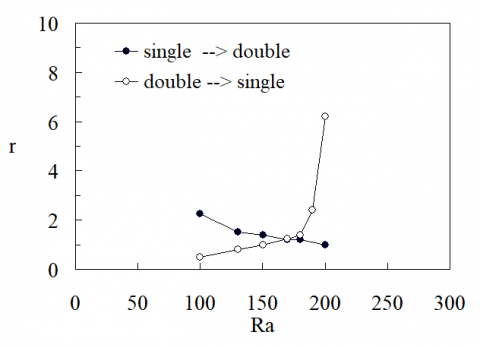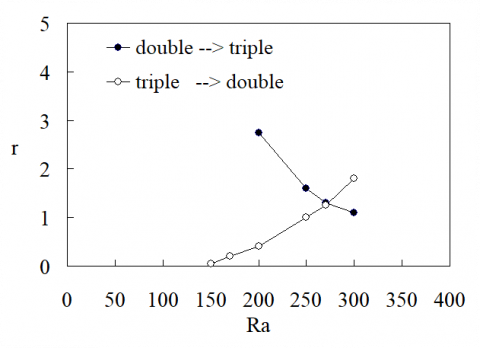# ON REALIZABLE CONVECTION PATTERNS IN A SATURATED POROUS SQUARE SECTION

ON REALIZABLE CONVECTION PATTERNS IN A SATURATED POROUS SQUARE SECTION

Kanazawa University, kakuma, Kanazawa, Ishikawa 920-1192

Corresponding Author Email:
skimura@se.kanazawa-u.ac.jp
Page:
S91-S94
|
DOI:
https://dx.doi.org/10.18280/ijht.34S111
| |
Accepted:
| | Citation

OPEN ACCESS

Abstract:

It is a well-known fact that there are several possible convecting patterns for a given Rayleigh number, when a saturated porous square section is heated from below and cooled at the top, with adiabatic side boundaries. The question is which pattern among them is selected preferably or naturally by the system for a fixed value of the Rayleigh number. A pseudo-spectral numerical method was employed in order to investigate the problem. There are two important findings. One is that the convecting pattern carrying the greatest heat from the bottom to the top boundaries is more stable against disturbances than other possible patterns. This finding is consistent with the Constructal law, as well as the Maximum entropy production hypothesis proposed by Malkus. The other one is that once the system has selected a certain pattern, it persists despite the more heat carrying pattern exists, unless sufficient disturbances are introduced into the system. The system exhibits a strong initial value dependency.

Keywords:

Convection, Porous media, Convection patterns, Heated from below, Preferred pattern selection.

1. Introduction

It is a very intriguing fact that there exist several convection patterns that are realizable in the fluid saturated porous layer, when it is subject to bottom heating and top cooling thermal boundary conditions, with the same geometric and heating rate (or cooling rate) conditions [1,2]. Now the immediate question that comes to our mind is if there is a preferred mode among several possible convection patterns for a given geometric condition and a given set of thermal boundary conditions. Malkus  claimed that, among many possible convection patterns in the clear fluid layer, the system naturally choses the one which can carry the heat most. This hypothesis is often termed as the entropy production maximum, since for the given top and bottom boundary temperatures, the maximum heat flux condition is equivalent to the maximum entropy production in the system. The research interest has been mounted on this problem in connection with geophysical fluid dynamics, where the atmospheric circulation takes a particular flow pattern among many possible ones [4, 5]. Are they truly stable in a time-scale of large enough comparable with the earth’s environmental evolution, i.e. global climate change? In order to make our discussion simple in this paper, however, we restrict ourselves to two-dimensional square cross section of saturated porous medium, with the Darcy flow model and the Boussinesq approximation. Within this strict condition, the convection system is controlled by the single non-dimensional parameter, the Rayleigh number . In the present paper, we numerically investigate about relative realizability among possible convection patterns for the given values of the Rayleigh number.

2. Description of Mathematical Model

The detailed physical assumptions made and the associated mathematical formulation are the same as those described in papers [2, 7]. Therefore, we will not repeat them all here. However, it should be noted that the Darcy flow model, the thermal equilibrium between the porous matrix and the saturating fluid, and the Boussinesq approximation for the buoyant force are assumed. In this case, referring to Fig. 1, it is possible to introduce a potential $\phi$, whose derivative with respect to $\xi$ is equivalent to the two-dimensional stream function. The Darcy’s law and the energy equation can be combined to yield the following single equation for the potential $\phi$.

$\frac{\partial}{\partial t} \nabla^{2} \phi+\phi_{\zeta \xi} \nabla^{2} \phi_{\xi}-\phi_{\xi \xi} \nabla^{2} \phi_{\zeta}=\nabla^{4} \phi+R a \phi_{\xi \xi}$(1)

The impermeable boundary conditions, adiabatic sidewalls and isothermal top and bottom temperature conditions are

$\phi_{\xi \xi}=\phi_{\zeta \zeta}=0(\zeta=0,1), \phi_{\xi \zeta}=\nabla^{2} \phi_{\xi}=0(\xi=0,1)$(2)

where Ra is the Rayleigh number defined in porous media

$R a=\frac{g \beta \Delta T K d}{\alpha v}$(3)

where the symbols have the usual meanings in porous media convection. The dimensionless quantities were introduced based on the size of the cross section and the diffusion time,

$(\xi, \zeta)=(x / d, z / d),$ and $t=\alpha \tau / d^{2}$(4)

We employ the Galerkin technique to solve for the potential $\phi$, satisfying the boundary conditions eq.(2). The solution can be expressed with the following Fourier-series expansion,

$\phi=\sum_{n=1}^{\infty} \sum_{j=0}^{\infty} \Phi_{n j}(t) \sin n \pi \zeta \cos j \pi \xi$(5)

Because of the purpose of the present paper, the numerical results are characterized by the average Nusselt number, i.e. the non-dimensional averaged heat flux,

$\overline{N u}=\frac{\overline{q}^{\prime \prime}}{k \Delta T / d}=1-\sum_{n=1}^{\infty} \frac{n^{3} \pi^{3}}{R a} \Phi_{n 0}(t)$(6)

In actual computations, the Fourier-series were truncated by an appropriate number N. The truncation number was determined by comparing the average Nusselt numbers with those reported in previous works [2,7]. It was found that N=64 is in fact large enough to reproduce the previously reported average Nusselt numbers within the Rayleigh number range less than 400.

3. Heat Fluxes of Different Modes of Convection

Nondimensional heat fluxes are summarized as the average Nusselt numbers in Fig. 2. It is generally clear that the higher modes of convection with multiple cells are capable of carrying greater heat fluxes than the lower modes at high Rayleigh numbers. For example, the double cell convection carries more heat than the single cell above Ra=150. The triple cell Nusselt number takes over that of double cell at Ra=300.

4. A Test of Relative Stability Among Different Modes of Convection

According to the linear stability analysis, six different modes of convection are possible in a range of the Rayleigh number up to 500 in a square cross section, among them we concern with the first three modes, i.e. single cell, double cell and triple cell patterns. In order to quantify the robustness of the respective patterns against another, we introduce numerical disturbances, and then observe how the system responds to the external excitements, i.e. if the disturbances decay, or grow steadily and eventually transform the current convecting mode into another. Probably there are several ways regarding how the disturbances can be introduced in the system. In the present work, after steady state with a particular mode of convection has been reached, we add a certain amount of disturbance, on the Fourier coefficient of $\Phi_{n j}$, representing a possibly realizable another mode. If the system is easily susceptible to the disturbance, the magnitude of the added disturbance on the Fourier coefficient can be small in order to transform the system to another mode. On the contrary, if the system is robust to the added disturbance, a significantly large magnitude of disturbance needs to be introduced. In this way, we are able to quantify the relative stability of a particular mode of convection against another mode. In the present paper, we are concerned with relative stabilities among the first three different convecting modes.

Fig. 3 shows how the relative stabilities of single cell convection mode against double cell convection mode vary in the course of increasing the Rayleigh number. The ordinate axis indicates the magnitude of disturbance introduced on the Fourier coefficient, representing the mode to which the system may be transformed. The magnitude of introduced disturbance is calculated by $r \Phi_{21}$, when we test the stability of single cell against double cell. A descending line indicates the boundary at which the introduced disturbance on the Fourier coefficient of the double mode can transform the system from single mode to double mode. The descending trend proves that the system (a single cell mode) becomes more and more susceptible to the added disturbances on the double mode as the Rayleigh number increases. On the contrary, the double cell convection becomes more and more robust against the single cell disturbances as the Rayleigh number increases. Since the double cell convection has higher Nusselt number than the single cell in the region of the Rayleigh number above 150, it can be said that the convection mode of higher heat flux is more robust against the external disturbances than that of less heat flux. The same trend is found among the double and the triple cells in Fig. 4.

5. Concluding Remarks

Different modes of convection pattern in a fluid-saturated porous square cross section, heated from below and cooled at the top, with insulating side boundaries have been studied numerically. Introducing the external artificial disturbances on a particular Fourier coefficient, the relative robustness of a particular convection mode has been determined against the other possible one. Since the single cell convection starts losing its stability easily against the double mode as the Rayleigh number becomes large, where the double cell can carry higher heat fluxes, it can be concluded that the convecting system with higher heat fluxes is more stable than that carrying less heat fluxes, when the both modes are realizable in the system. The same trend is found between double and triple cells. It is also noted that once a particular mode of pattern is established in the system, it continues to exist unless a sufficiently large external disturbance is introduced, regardless of relative magnitude of heat fluxes between the two competing modes.

## 1.pngFigure 1. Saturated porous medium square cross section, the coordinates and thermal boundary conditions. All boundaries are impermeable to the saturating fluid

## 2.pngFigure 2. Average Nusselt numbers of different modes of convection as a function of Ra

## 3.pngFigure 3. Relative robustness between single and double cells, against externally exerted disturbances. The ordinate r indicates the relative magnitudes of disturbance added on the representative Fourier coefficient, to which we want to transform the system

## 4.pngFigure 4. Relative robustness between double and triple cells at different Rayleigh numbers

References

1. Caltagirone, J.P., “Thermoconvective instabilities in a horizontal porous layer,” J. Fluid Mech., 72(2), 269-287, 1975. DOI: 10.1017/S0022112075003345.

2. Schubert, G., Straus, J.M., “Three-dimensional and multicellular steady and unsteady convection in fluid-saturated porous media at high Rayleigh number,” J. Fluid Mech., 94(1), 25-38, 1979. DOI: 10.1017/S0022112079000926.

3. Malkus W.V.R., Veronis, G., “Finite amplitude cellular convection,” J. Fluid Mech., 4(3), 225-260, 1979. DOI: 10.1017/S0022112079000926.

4. Nelson, Jr. R.A., Bejan, A., “Constructal optimization of internal flow geometry in convection,” J. Heat Transfer, 120, 357-364, 1998. DOI: 10.1115/1.2824257.

5. Ozawa, H., Ohmura, A., Lorenz, R.D., Pujol, T., “The second law of thermodynamics and the global climate system: a review of the maximum entropy production principle,” Review of Geophysics, 41(4), 1-24, 2003. DOI: 10.1029/2002RG000113.

6. Nield, D.A, Bejan, A., Convection in Porous Media, 4th edition, 2013, Springer, New York.

7. Kimura, S., Schubert, G., Straus, J.M., “Route to chaos in porous-medium thermal convection,” J. Fluid Mech. 166, 305-324, 1986. DOI: 10.1017/S0022112086000162.

8. Bejan, A., Advanced Engineering Thermodynamics, 3rd edition, 2006, John-Wiley & Sons.

9. Bejan, A., Peder Zane, J., Design in Nature, 2012, Doubleday, New York.